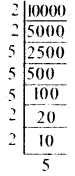# KSEEB Solutions for Class 6 Maths Chapter 3 Playing with Numbers Ex 3.5

Students can Download Chapter 3 Playing with Numbers Ex 3.5 Questions and Answers, Notes Pdf, KSEEB Solutions for Class 6 Maths helps you to revise the complete Karnataka State Board Syllabus and score more marks in your examinations.

## Karnataka State Syllabus Class 6 Maths Chapter 3 Playing with Numbers Ex 3.5

Question 1.
Which of the following statements are true?
a) If a number is divisible by 3, it must be divisible by 9.
b) If a number is divisible by 9, it must be divisible by 3.
c) A number is divisible by 18, if it is divisible by both 3 and 6.
d) If a number is divisible by 9 and 10 both, then it must be divisible by 90.
e) If two numbers are co-primes, at least one of them must be prime.
f) All numbers which are divisible by 4 must also be divisible by 8.
g) All numbers which are divisible by 8 must also be divisible by 4.
h) If a number exactly divides two numbers separately, it must exactly divide their sum.
i) If a number exactly divides the sum of two numbers, it must exactly divide the two numbers separately.
Solution:
(a) False
6 is divisible by 3, but not by 9.

(b) True, as 9 = 3 × 3
Therefore, if a number is divisible by 9, then it will also be divisible by 3.

(c) False
30 is divisible by 3 and 6 both, but it is not divisible by 18.

(d) True, as 9 × 10 = 90
Therefore, if a number is divisible by 9 and 10 both, then it will also be divisible by 90.

(e) False
15 and 32 are co-primes and also composite.(f) False
12 is divisible by 4, but not by 8.

(g) True, as 8 = 2 × 4
Therefore, if a number is divisible by 8, then it will also be divisible by 2 and 4.

(h) True
2 divides 4 and 8 as well as 12. (4 + 8 = 12)

(i) False
2 divides 12, but does not divide 7 and 5.

Question 2.
Here are two different factor trees for 60. Write the missing numbers.
Solution:Question 3.
Which factors are not included in the prime factorisation of a composite number ?
Solution:
1 and the number itself.Question 4.
Write the greatest 4-digit number and express it in terms of its prime factors.
Solution:
Greatest four-digit number = 9999
9999 = 3 × 3 × 11 × 101Question 5.
Write the smallest 5-digit number and express it in the form of its prime factors.
Solution:10,000
= 2 × 2 × 2 × 2 × 5 × 5 × 5 × 5

Question 6.
Find all the prime factors of 1729 and arrange them in ascending order. Now state the relation, if any; between two consecutive prime factors.
Solution:
1729 = 7 × 13 × 19
13 – 7 = 6, 19 – 13 = 6The difference of two consecutive prime factors is 6.

Question 7.
The product of three consecutive numbers is always divisible by 6. Verify this statement with the help of some examples.
Solution:
2 × 3 × 4 = 24, which is divisible by 6
9 × 10 × 11 = 990, which is divisible by 6
20 × 21 × 22 = 9240, which is divisible by 6

Question 8.
The sum of two consecutive odd numbers is divisible by 4. Verily this statement with the help of some examples.
Solution:
3 + 5 = 8, which is divisible by 4
15 + 17 = 32, which is divisible by 4
19 + 21 = 40, which is divisible by 4

Question 9.
In which of the following expressions, prime factorisation has been done?
(a) 24 = 2 × 3 × 4
(b) 56 = 7 × 2 × 2 × 2
(c) 70 = 2 × 5 × 7
(d) 54 = 2 × 3 × 9
Solution:
a) 24 = 2 × 3 × 4
Since 4 is composite, prime factorisation has not been done.

(b) 56 = 7 × 2 × 2 × 2
Since ail the factors are prime, prime factorisation has been done.

(c) 70 = 2 × 5 × 7
Since all the factors are prime, prime factorisation has been done.

(d) 54 = 2 × 3 × 9
Since 9 is composite, prime factorisation has not been done.

Question 10.
Determine if 25110 is divisible by 45.
|Hint: 5 and 9 are co-prime numbers. Test the divisibility of the number by 5 and 9|.
Solution:
45 = 5 × 9
Factors of 5 = 1, 5
Factors of 9 = 1, 3, 9
Therefore, 5 and 9 are co-prime numbers. Since the last digit of 25110 is 0, it is divisible by 5.
Sum of the digits of 25110 = 2 + 5 + 1 + 1 + 0 = 9
As the sum of the digits of 25110 is divisible by 9. therefore, 25110 is divisible by 9.
Since the number is divisible by 5 and 9 both, it is divisible by 45.

Question 11.
18 is divisible by both 2 and 3. It is also divisible by 2 × 3 = 6. Similarly, a number is divisible by both 4 and 6. Can we say that the number must also be divisible by 4 × 6 = 24? If not, give an example to justify your answer.
Solution:
No. It is not necessary because 12 and 36 are divisible by 4 and 6 both, but are not divisible by 24.Question 12.
I am the smallest number, having four different prime factors. Can you find me?
Solution:
Since it is the smallest number of such type, it will be the product of 4 smallest prime numbers.
2 × 3 × 5 × 7 = 210

error: Content is protected !!